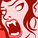# QlikView App Dev

Discussion Board for collaboration related to QlikView App Development.

Announcements
QlikWorld 2022, LIVE in Denver CO., May 16-19, 2022. REGISTER NOW TO RECEIVE EARLY BIRD PRICING
cancel
Showing results for
Did you mean:Contributor III

## Help with variable within set analysis

Hello,

Example of current set analysis:

=num(sum({\$<Lev = {1},PeriodCounter ={">=\$(#=max(PeriodCounter)-11<=\$(#=max(PeriodCounter))"},period=>}Netsales)*-1/1000000,'# ##0')

I want to store the red part (rolling 12) in a variable. But how do I then use that variable correct? I can't get the right syntax.

Thanks,

Meg

1 Solution

Accepted SolutionsPartner

Hi!

You should create the variable vSetExpression with the following content

PeriodCounter ={">=\$(#=max(PeriodCounter)-11<=\$(#=max(PeriodCounter))"},period=

And then you invoque it as follows

=num(sum({\$<Lev = {1},\$(vSetExpression )>}Netsales)*-1/1000000,'# ##0')

Let me know your doubts! Regards,

Jaime.

2 RepliesPartner

Hi!

You should create the variable vSetExpression with the following content

PeriodCounter ={">=\$(#=max(PeriodCounter)-11<=\$(#=max(PeriodCounter))"},period=

And then you invoque it as follows

=num(sum({\$<Lev = {1},\$(vSetExpression )>}Netsales)*-1/1000000,'# ##0')

Let me know your doubts! Regards,

Jaime.Contributor III
Author

Thank you, was more simple than I thought:)Community Browser